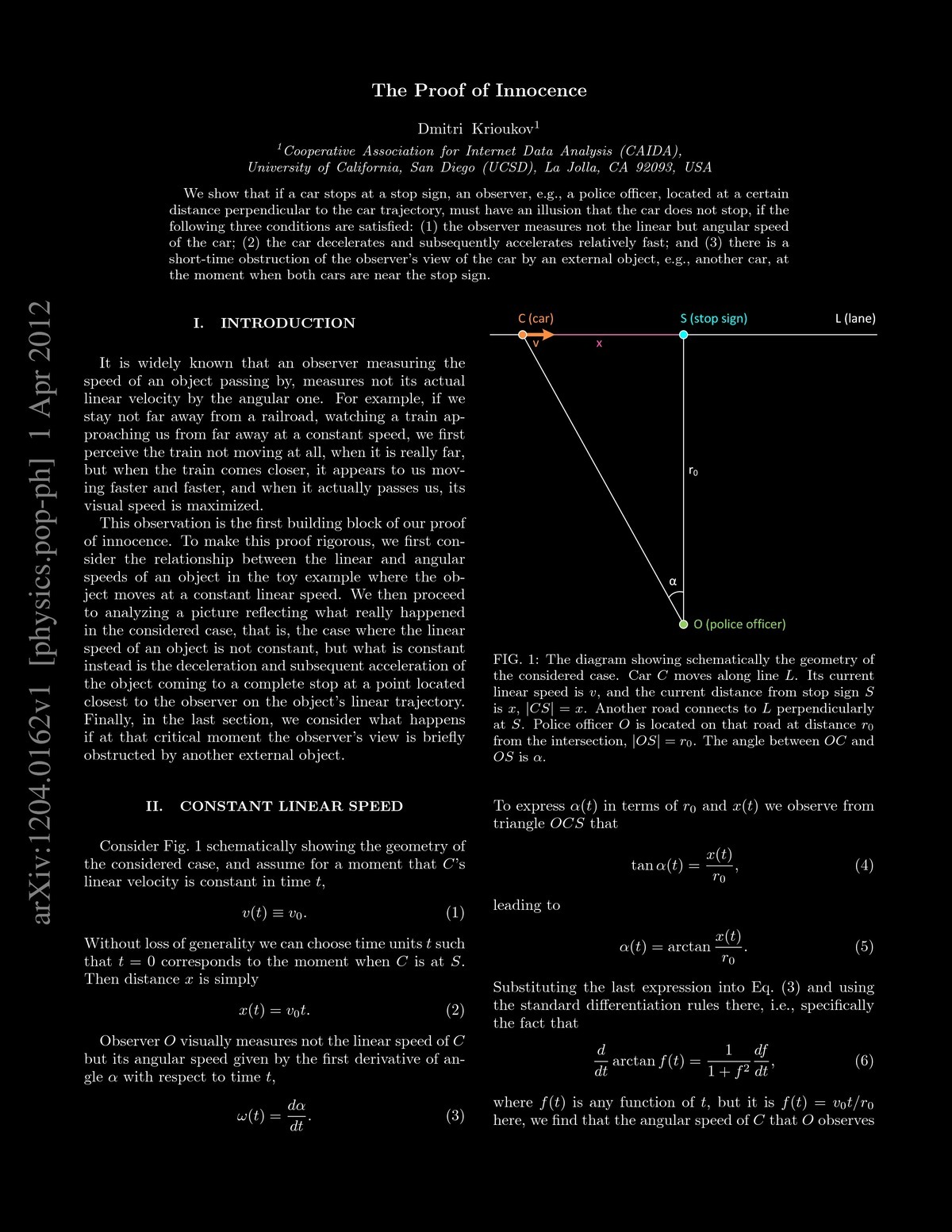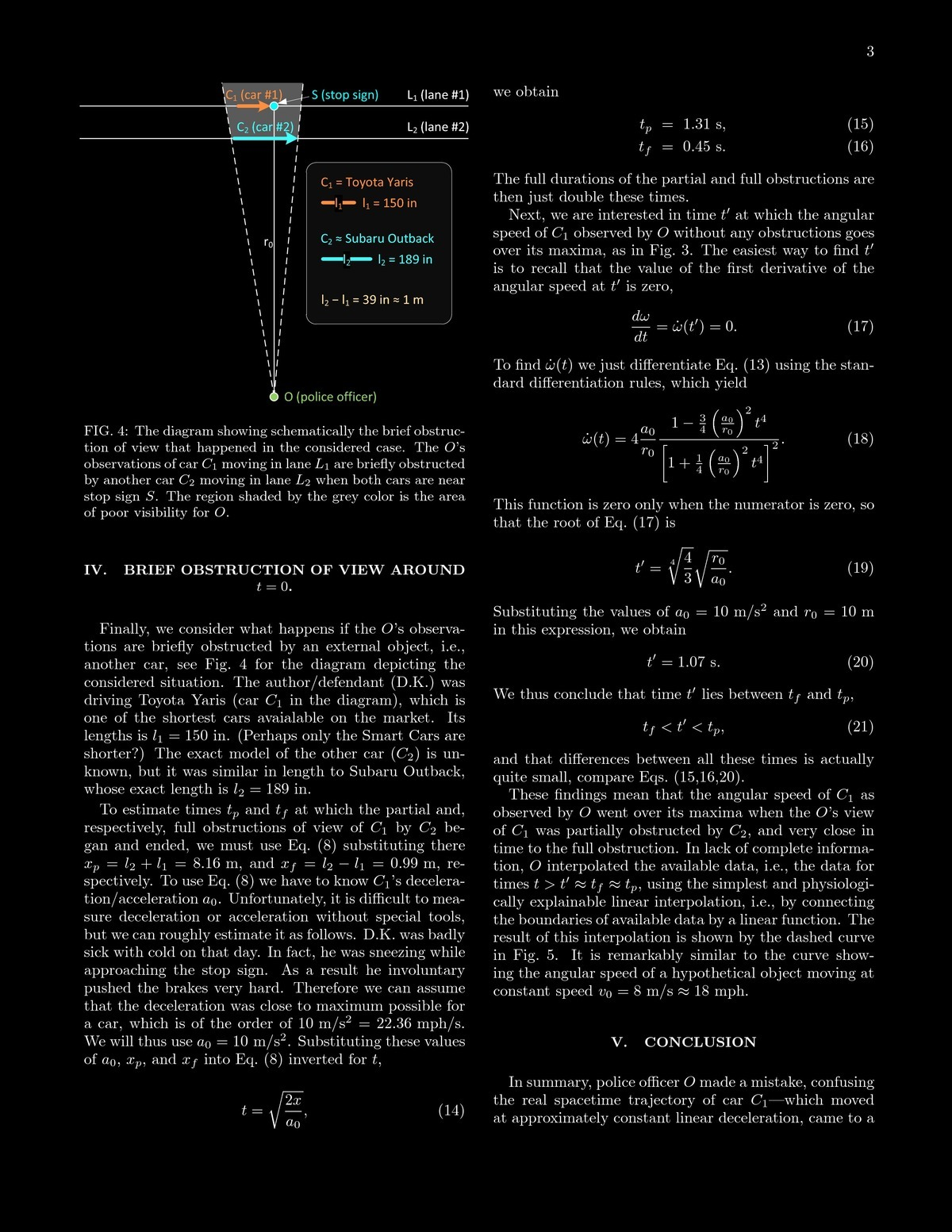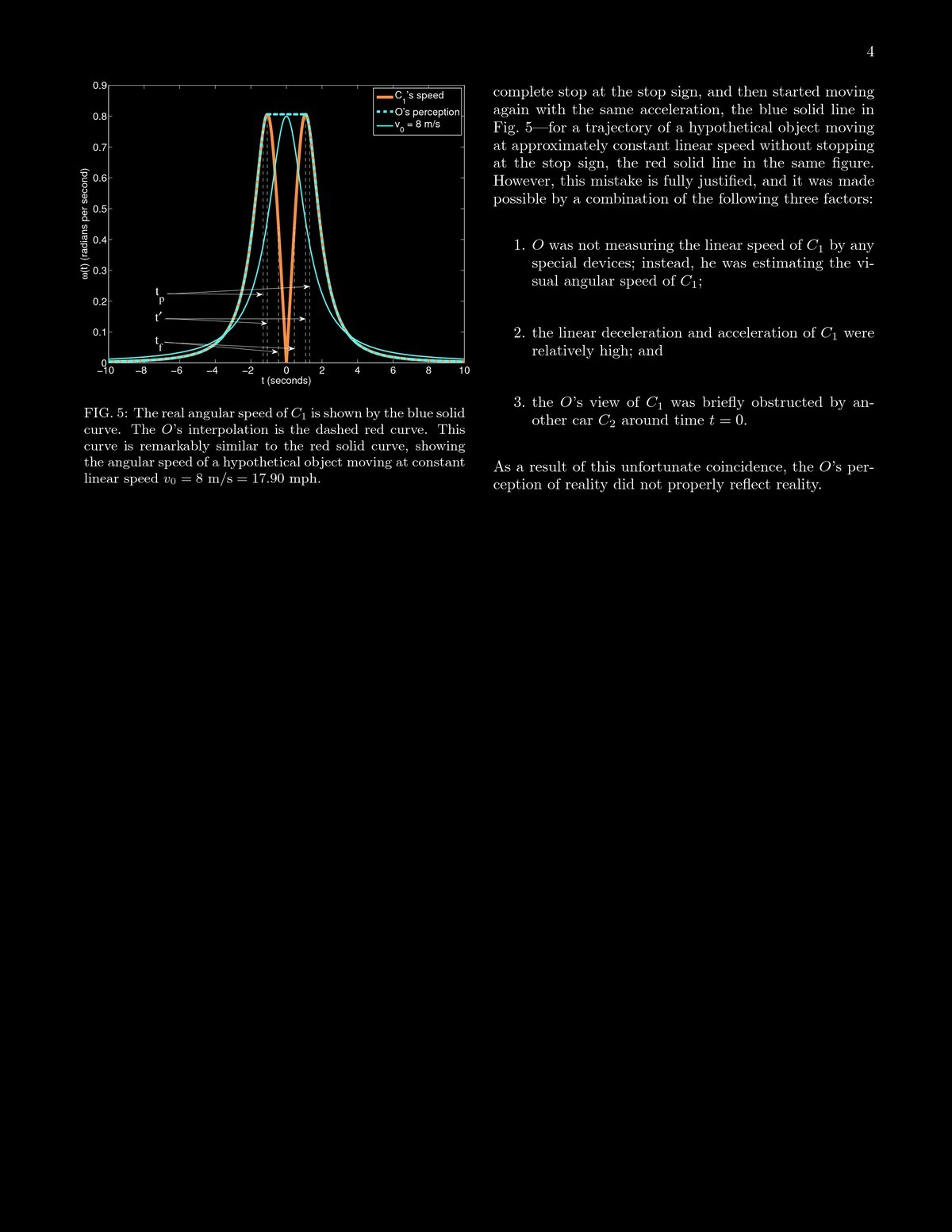# Witch of Agnesi beats traffic ticket

Knowing some math can help you avoid a ticket. A car approaches a stop signs and runs it with constant speed. A policemen observes this. How does the angular velocity change? This is a related rate problem we can solve (remember the airplane prloblem in the homework). Assume the policemen is at distance r from the stop sign. Since x = r tan(a) we get a=arctan(x/r) and a'=(v/r)/(1+(x(t)^2/r^2). This function is the "witch of Agnesi" which is also important in statistics when we look at the Cauchy distribution.

PDF (Source)src="i1001.jpg" width="100%">